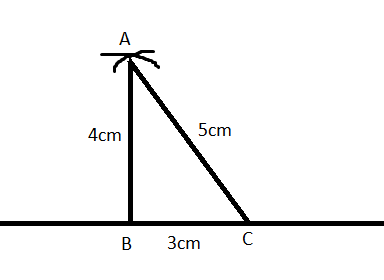QUESTION

# Construct an isosceles triangle whose perimeter is 12 centimetre and altitude 4 cm.

Hint: For solving this problem, use the data given in the problem statement. By using the parameter and the altitude, we proceed by drawing on a line segment obtained from the problem.

Complete Step-by-Step solution:
According to the question, we are given the perimeter of a triangle as 12 cm. Now, by using the ratio provided, we calculate the length of each side. Mathematically, it can be expressed as:
Perimeter of triangle ABC = 12cm
Ratio of the sides $=3:4:5$
As, perimeter is the sum of all the sides. Now, let the common quantity inside be x. So, by using the ratio, we get
\begin{align} & 3x+4x+5x=12 \\ & 12x=12 \\ & x=1 \\ \end{align}
Therefore, length of AB = 3 cm, BC = 4 cm and AC = 5 cm.
Now, for constructing the triangle we follow the below given steps:
(i) Draw a line segment using a ruler on a plane sheet of paper.
(ii) Locate points B and C on the line segment such that BC=3 cm.
(iii) With B as centre and radius 4cm, draw an arc.
(iv) With C as centre and radius 5cm, draw another arc cutting the previous arc at C.
(v) Join AC and AB to get the desired triangle.Note: The key concept for solving this problem is the knowledge of the perimeter of a triangle. Once we obtain the length of each side, we can easily construct the triangle using geometrical tools. This knowledge is useful for solving complex problems.